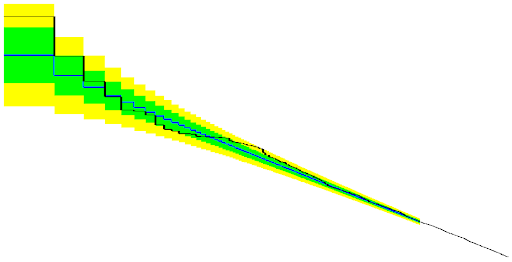## Monday, March 19, 2012

### Should one be shocked by several high-energy events?

Technical: If you see a nation-specific URL different from motls.blogspot.com, you won't be able to read other people's fast comments or post yours in a visible way. To fix that, click at the big "the reference frame" title at the top which brings you to motls.blogspot.com/ncr, the canonical American edition of this blog. Ncr stands for "no country redirect" and must be added right after ".com/". Regular readers in these countries may want to manually edit the bookmarks' URL to contain ".com/ncr".

During three recent days, I've gone through dozens of PDF files with talks from Search 2012, a workshop in Maryland. No obvious signal of new physics has been announced. I still liked many events in the tails, like this 2.4 TeV event with a muon and a neutrino in this talk.University of Maryland, campus

Very often, I am looking – and I am sure that many actual professional particle physicists are looking – at various exclusion charts with many bins. The expected number of events dramatically decreases for high energy (whether it is the effective mass or some invariant mass of a few particles etc. on the x-axis) and there are a few events with a very high energy that belong to the tail of the distribution. Their number and/or their energy looks a bit higher than one would expect and many of us would surely love to know how much special those events and their small collectives are.

See the ATLAS 1,600 GeV events for a recent example.

I have developed a set of conventions to address all these questions and in my opinion, many such measurements should be reorganized into these new K-S graphs; I've chosen this modest name for the Motl graphs because Tommaso Dorigo told me about related Kolmogorov-Smirnov tests in statistics.

How does it work?

It's simple. You calculate (in some way) your theoretically predicted probability distribution for the events as a function of energy $$E$$. It may be $$m_\text{eff}$$ or $$m_\text{invariant}$$ or another kind of mass or energy that is represented by the $$x$$-axis of some graphs whose $$y$$-axis tells us about the number of events in individual bins.

Now, you use this theoretically predicted PDF (probability distribution function) to calculate the expected energy $$E_n$$ of the $$n$$-th highest-energy event in the sample. You calculate the 1-sigma, 2-sigma, and 3-sigma bands around this $$E_n$$ as well. I am confident that all these things may be fully computed from the original statistical distribution and nothing else. The process of calculation requires you to compute the CDF, the cumulative probability function (the integral of the PDF) and its inverse.

You draw the K-S chart. On the $$x$$-axis, you have $$\log(n)$$ and the bar between $$\log(n)$$ and $$\log(n+1)$$ represents the energy of the $$n$$-th highest-energy event in the sample. A black curve depicts the actual energy of the $$n$$-th highest-energy measured event while the Brazil bands (perhaps with another, 3-sigma colorful band) tell you about the expectations. A large, 3-sigma etc. deviation for any $$x$$ i.e. the order of the event's energy, represents an interesting signal. Obviously, you can't add the statistical significance of nearby columns because they're not independent.

The resulting graph may look like this:Click to zoom in and imagine that $$\log(n)$$ is on the $$x$$-axis while energy $$E$$ is on the $$y$$-axis.
Get the PDF printout of the Mathematica notebook that calculated those things...
This is an example for 1000 events in a very simple distribution – an exponentially decaying one. The big rectangular box on the left side is the energy of the highest-energy event. The blue level in the middle is the theoretically predicted mean value of the highest-energy event. The green and yellow (i.e. Brazil) bands are the usual 1-sigma and 2-sigma intervals. The second smaller box is for the second highest-energy event, and so on, up to the 1000th highest-energy event on the right side (where they become dense).

You see that there's a 2-sigma bump somewhere in the middle of the picture. It would be otherwise hard to see that it's 2 sigma. I must tell you what I did. I randomly generated the "experimental" collisions – energies in the distribution decaying as $$\exp(-E/800\GeV)$$ – but I contaminated those 1000 collisions by 10 "new physics" events randomly spread between 3,000 and 3,300 GeV. The test was able to see the deviation.

Tommaso Dorigo is essentially telling me that one can't usually determine the statistical confidence of the events in the tail anyway because the theoretical prediction of these tail events is usually known very inaccurately. That's too bad. Did you notice the "teeth" in many graphs of the theoretical predictions? They're produced by Monte Carlo software which wasn't repeated sufficiently many times. Independently of that, it's sad that people don't have enough CPU time to make more accurate predictions over there.

In fact, I believe that a more clever software could estimate the high-energy behavior of the predicted cross sections – which is often close to a power law – and a "brute force Monte Carlo" combined with some smarter and more analytical method could yield better predictions. Well, I think that despite the folks' very high intelligence, many methods still indicate a rather primitive state of the HEP-EX affairs.

The visualization technique above has many advantages. For example, it depends on no parameters such as the width of a bin. The bands are in the energy direction, not the "number of events" direction, and for uniformly decaying cross sections as a function of energy, these energy bands are pretty uniformly thick. (They're not uniform for other, more extreme cases.)

LHC running at 4 TeV per beam

The LHC is already circulating beams of energy 4 TeV (twice). Their collisions will begin around April 7th.